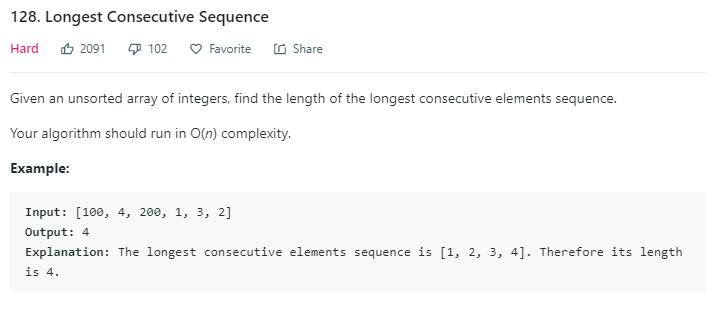# 题目描述（困难难度）# 解法一

public int longestConsecutive(int[] nums) {
HashSet<Integer> set = new HashSet<>();
for (int i = 0; i < nums.length; i++) {
}
int max = 0;
for (int i = 0; i < nums.length; i++) {
int num = nums[i];
int count = 0;
while (set.contains(num)) {
count++;
num += 1;
}
max = Math.max(max, count);
}
return max;
}


public int longestConsecutive(int[] nums) {
HashSet<Integer> set = new HashSet<>();
for (int i = 0; i < nums.length; i++) {
}
int max = 0;
for (int i = 0; i < nums.length; i++) {
int num = nums[i];
//n - 1 是否存在
if (!set.contains(num - 1)) {
int count = 0;
while (set.contains(num)) {
count++;
num += 1;
}
max = Math.max(max, count);
}
}
return max;
}


# 解法二

假如我们已经了有连续的序列，123 和 56，并且序列的边界保存了当前序列的长度。
1  2  3
3     3  <- 序列长度

5  6
2  2  <- 序列长度

1  2  3  4  5  6
3     3     2  2   <- 序列长度

1  2  3  4  5  6
6     3     2  6   <- 序列长度

1  2  3  4  5  6  7
6     3     2  6     <- 序列长度

1  2  3  4  5  6 7
7     3     2  6 7  <- 序列长度


public int longestConsecutive(int[] nums) {
HashMap<Integer, Integer> map = new HashMap<>();
int max = 0;
for (int i = 0; i < nums.length; i++) {
int num = nums[i];
//已经考虑过的数字就跳过，必须跳过，不然会出错
//比如 [1 2 1]
//最后的 1 如果不跳过，因为之前的 2 的最长长度已经更新成 2 了，所以会出错
if(map.containsKey(num)) {
continue;
}
//找到以左边数字结尾的最长序列，默认为 0
int left = map.getOrDefault(num - 1, 0);
//找到以右边数开头的最长序列，默认为 0
int right = map.getOrDefault(num + 1, 0);
int sum = left + 1 + right;
max = Math.max(max, sum);

//将当前数字放到 map 中，防止重复考虑数字，value 可以随便给一个值
map.put(num, -1);
//更新左边界长度
map.put(num - left, sum);
//更新右边界长度
map.put(num + right, sum);
}
return max;
}